JEE  >  BITSAT Logical Reasoning Test - 1

# BITSAT Logical Reasoning Test - 1

Test Description

## 10 Questions MCQ Test BITSAT Mock Tests Series & Past Year Papers | BITSAT Logical Reasoning Test - 1

BITSAT Logical Reasoning Test - 1 for JEE 2022 is part of BITSAT Mock Tests Series & Past Year Papers preparation. The BITSAT Logical Reasoning Test - 1 questions and answers have been prepared according to the JEE exam syllabus.The BITSAT Logical Reasoning Test - 1 MCQs are made for JEE 2022 Exam. Find important definitions, questions, notes, meanings, examples, exercises, MCQs and online tests for BITSAT Logical Reasoning Test - 1 below.
Solutions of BITSAT Logical Reasoning Test - 1 questions in English are available as part of our BITSAT Mock Tests Series & Past Year Papers for JEE & BITSAT Logical Reasoning Test - 1 solutions in Hindi for BITSAT Mock Tests Series & Past Year Papers course. Download more important topics, notes, lectures and mock test series for JEE Exam by signing up for free. Attempt BITSAT Logical Reasoning Test - 1 | 10 questions in 10 minutes | Mock test for JEE preparation | Free important questions MCQ to study BITSAT Mock Tests Series & Past Year Papers for JEE Exam | Download free PDF with solutions
 1 Crore+ students have signed up on EduRev. Have you?
BITSAT Logical Reasoning Test - 1 - Question 1

### Choose the pair in which the words are differently related.

Detailed Solution for BITSAT Logical Reasoning Test - 1 - Question 1

In all other pairs, second is a part of the first.

BITSAT Logical Reasoning Test - 1 - Question 2

### Complete the analogous pair. Command : Order : : Confusion : ?

Detailed Solution for BITSAT Logical Reasoning Test - 1 - Question 2

The word in each pair are synonyms.

BITSAT Logical Reasoning Test - 1 - Question 3

### Find the missing character.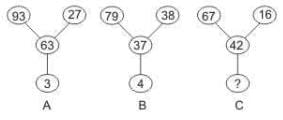Detailed Solution for BITSAT Logical Reasoning Test - 1 - Question 3

In A, 93 – (63 + 27) = 3
In B, 79 – (37 + 38) = 4

By sensing the pattern:
In C, 67 – (42 + 16) = 9

BITSAT Logical Reasoning Test - 1 - Question 4

Find out the term in the number series which is wrong.
1, 2, 4, 8, 16, 32, 64, 96

Detailed Solution for BITSAT Logical Reasoning Test - 1 - Question 4

Each term of the series is obtained by multiplying the preceding term by 2.

So, 96 is wrong and must be replaced by (64 * 2)  i.e. 128

BITSAT Logical Reasoning Test - 1 - Question 5

Find out the term in the number series which is wrong.
89, 78, 86, 80, 85, 82, 83

Detailed Solution for BITSAT Logical Reasoning Test - 1 - Question 5

Take even place numbers: 78, 80, 82

78 - 80 = 2
80 - 82 = 2

Take odd place numbers: 89, 86, 85, 83

89 - 86 = 3
86 - 85 = 1
85 - 83 = 2

If we change the number 87 instead of 86 we will get the difference are 2, 2, 2.
So, the odd term is 86.

BITSAT Logical Reasoning Test - 1 - Question 6

Group the following figures into three classes on the basis of identical properties.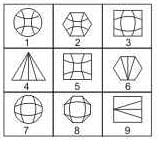Detailed Solution for BITSAT Logical Reasoning Test - 1 - Question 6

By sensing the pattern by the internal design in the figure.

Groups formed:
1, 2, 5
3, 7, 8
4, 6, 9

BITSAT Logical Reasoning Test - 1 - Question 7

Select the correct mirror-image of the Figure (X) from amongst the given alternatives.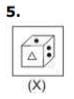Detailed Solution for BITSAT Logical Reasoning Test - 1 - Question 7

Because in mirror image right becomes left and left becomes right.

BITSAT Logical Reasoning Test - 1 - Question 8

Select the correct mirror-image of the Figure (X) from amongst the given alternatives.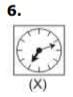Detailed Solution for BITSAT Logical Reasoning Test - 1 - Question 8

Because in mirror image right becomes left and left becomes right.

BITSAT Logical Reasoning Test - 1 - Question 9

Each of the following questions consists of five Problem figures followed by five Answer figures marked A, B, C, D, and E . Select a figure from the Answer Figures which will continue the same series as given in the Problem figures.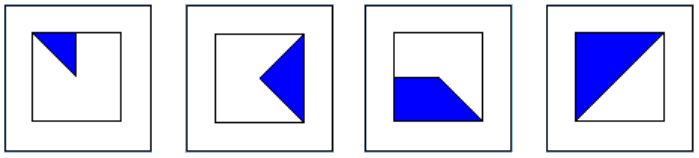Detailed Solution for BITSAT Logical Reasoning Test - 1 - Question 9

Each box increments by one blue segment (one eight of the square). So there are 4 segments in box four (half the square is filled).
So there must be 5 blue segments (5/8th of the square) in the answer which leaves us with A or D.
But the diagonal edge of the first segment is also rotating clockwise by 90% each time, so it should be aligned NW in the answer.

BITSAT Logical Reasoning Test - 1 - Question 10

Each of the following questions consists of five Problem figures followed by five Answer figures marked A, B, C, D, and E . Select a figure from the Answer Figures which will continue the same series as given in the Problem figures.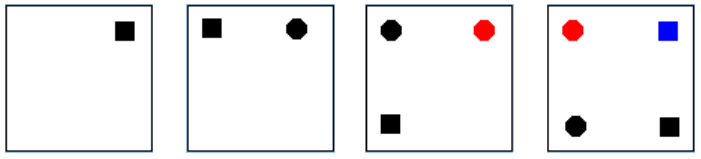Detailed Solution for BITSAT Logical Reasoning Test - 1 - Question 10

Items are introduced in this order: black square, black dot, red dot, blue square, green dot. New items are always introduced into the top right corner.

## BITSAT Mock Tests Series & Past Year Papers

3 videos|15 docs|70 tests
 Use Code STAYHOME200 and get INR 200 additional OFF Use Coupon Code
Information about BITSAT Logical Reasoning Test - 1 Page
In this test you can find the Exam questions for BITSAT Logical Reasoning Test - 1 solved & explained in the simplest way possible. Besides giving Questions and answers for BITSAT Logical Reasoning Test - 1, EduRev gives you an ample number of Online tests for practice

## BITSAT Mock Tests Series & Past Year Papers

3 videos|15 docs|70 tests